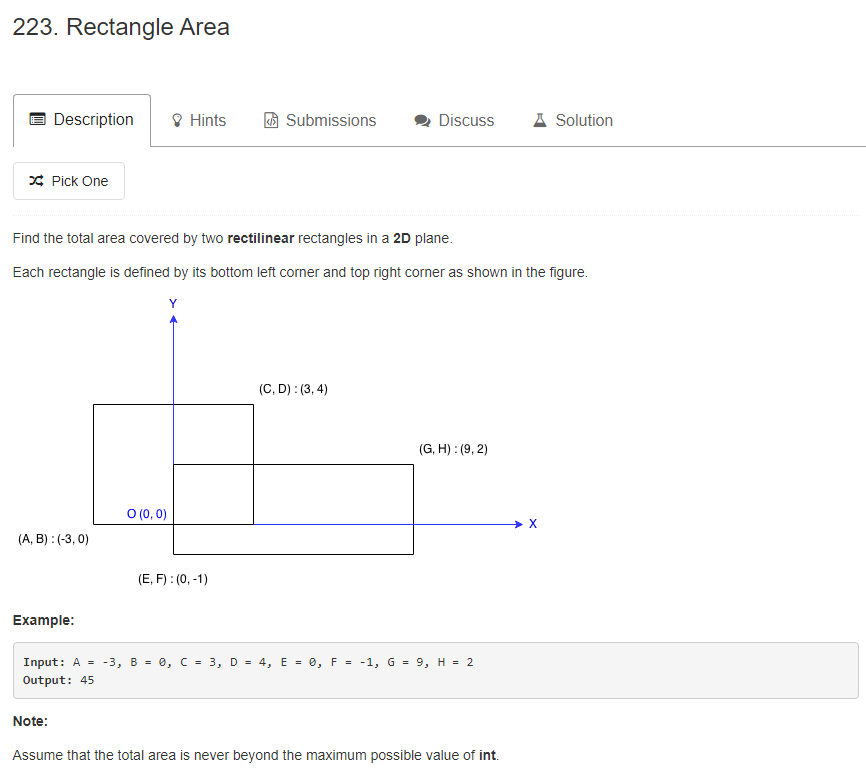# 题目描述（中等难度）# 解法一

public int computeArea(int A, int B, int C, int D, int E, int F, int G, int H) {
//求第一个矩形的面积
int length1 = C - A;
int width1 = D - B;
int area1 = length1 * width1;

//求第二个矩形的面积
int length2 = G - E;
int width2 = H - F;
int area2 = length2 * width2;

// 没有重叠的情况
if (E >= C || G <= A || F >= D || H <= B) {
return area1 + area2;
}

//确定右边
int x1 = Math.min(C, G);
//确定左边
int x2 = Math.max(E, A);
int length3 = x1 - x2;

//确定上边
int y1 = Math.min(D, H);
//确定下边
int y2 = Math.max(F, B);
int width3 = y1 - y2;
int area3 = length3 * width3;

return area1 + area2 - area3;
}